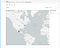# Sharing data between Node.js & Python cells

`%%nodevar wave = [];for (var i = 0; i < 1000; i++) {    var x = 2*Math.PI * i/ 360;    var obj = {      x: x,      i: i,      sin: Math.sin(x),      cos: Math.cos(x),      tan: Math.tan(x)    };    wave.push(obj);}`
`%%nodestore(wave, 'w');`
`# count of dataframeprint w.count()# maximum value of the sin waveprint w['sin'].max()# minimum value of the sin waveprint w['sin'].min()# average value of the tan waveprint w['tan'].mean()`

# Accessing a Cloudant database from a notebook

`npm.install('cloudant-quickstart')`
`%%node// connect to Cloudant using cloudant-quickstartvar cloudantqs = require('cloudant-quickstart');var cities = cloudantqs('https://reader.cloudant.com/cities');`

# Exploring the data using Node.js in a notebook

`%%nodecities.get('2636749').then(print);// {"name": "Stowmarket", "country": "GB", "longitude": 0.99774, "latitude": 52.18893, "timezone": "Europe/London", "_id": "2636749", "population": 15394}`
`%%nodecities.get(['4562407','2636749','3530597']).then(print);// [{"name": "York", "country": "US", "longitude": -76.72774, "latitude": 39.9626, "timezone": "America/New_York", "_id": "4562407", "population": 43718}, {"name": "Stowmarket", "country": "GB", "longitude": 0.99774, "latitude": 52.18893, "timezone": "Europe/London", "_id": "2636749", "population": 15394}, {"name": "Mexico City", "country": "MX", "longitude": -99.12766, "latitude": 19.42847, "timezone": "America/Mexico_City", "_id": "3530597", "population": 12294193}]`
`%%nodecities.get(['4562407','2636749','3530597']).then(display);`The cloudant-quickstart npm module querying a Cloudant database, from within the context of a Python Jupyter Notebook.
`%%node// fetch cities in UK above latitude 54 degrees northcities.query({country:'GB', latitude: { "\$gt": 54}}).then(display);`

# Aggregating data

`%%nodecities.sum('population').then(print);// 2694222973`
`%%nodecities.sum('population','country').then(print)// {"BD": 25149982, "BE": 7224564, "BF": 2381615, "BG": 4401796, "BA": 1790001 ....`
`%%nodecities.all({limit:25000}).then(function(data) {  store(data, 'y');});// y stored`
`y['population'].sum()# 2694222973`

Written by